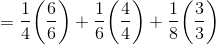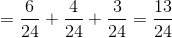# SSAT Upper Level Math : How to find the reciprocal of a fraction

## Example Questions

### Example Question #1 : How To Find The Reciprocal Of A Fraction

Give the reciprocal of 0.8.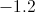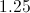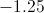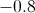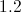Explanation:

The reciprocal of a number is the quotient of 1 and that number. Divide 1 by 0.8 by moving the decimal point to the right in each number one place: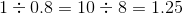### Example Question #2 : Reciprocals

Give the reciprocal of 0.75.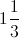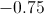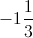Explanation:

The reciprocal of a number is the quotient of 1 and the number.

Divide 1 by 0.75 by moving the decimal point to the right in each number two places: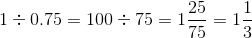### Example Question #3 : Reciprocals

Add the reciprocals of 0.3 and 0.7. What is their sum?

The correct answer is not among the other responses.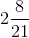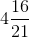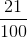Explanation: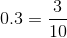, so its reciprocal is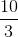.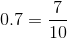, so its reciprocal is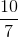.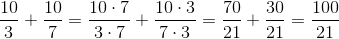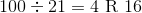, so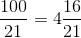### Example Question #4 : Reciprocals

Subtract the reciprocal of 1.5 from the reciprocal of 0.125. What is the result?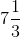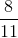The correct answer is not among the other responses.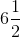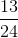Explanation: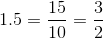, so its reciprocal is.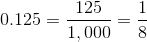, so its reciprocal is 8.

The requested difference is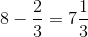### Example Question #5 : Reciprocals

Letbe the reciprocal of 0.22. Which of the following is true of?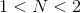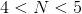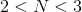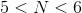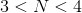Explanation: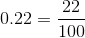, so its reciprocal is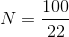.

Since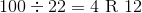,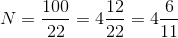Therefore,.

### Example Question #6 : Reciprocals

Letbe the reciprocal of 1.77. Which of the following is true of?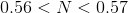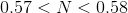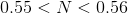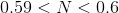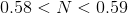Explanation: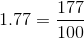, so its reciprocal is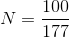.

If 100 is divided by 177, the decimal equivalent is seen to be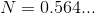so.

### Example Question #7 : Reciprocals

Subtract the reciprocal of 11 from the reciprocal of 13. What is the result?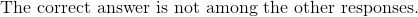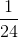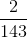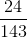Explanation:

The reciprocals of 11 and 13 are, respectively,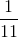and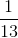. The requested difference is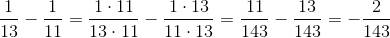This answer is not among the given choices.

### Example Question #8 : Reciprocals

Add the reciprocals of,, and. What is the sum?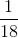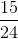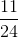Explanation:

The reciprocals of 4, 6, and 8 are, respectively,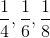. Their sum is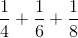First we find the least common denominator between all three fractions.